Annu. Rev. Astron. Astrophys. 1998. 36: 267-316 Copyright © 1998 by Annual Reviews. All rights reserved

2.2. Low Resolution Spectroscopy: Mean Absorption

The most basic observable of the Lyforest is the quantity originally sought by Gunn and Peterson (1965), the flux decrement D, or the mean fraction of the QSO continuum absorbed. It has become standard practice to measure the mean flux decrement DA between the Lyand Lyemission lines (Oke & Korycansky 1982):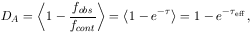(1)

where fobs is the observed (= residual) flux, fcont the estimated flux of the unabsorbed continuum, andis the resonance line optical depth as a function of wavelength or redshift. The absorption is measured against a continuum level usually taken to be a power law in wavelength extrapolated from the region redward of the Lyemission line. A knowledge of the mean absorption allows us, for example, to determine the resulting broadband color gradients in a QSO or high redshift galaxy spectrum, as caused by the Lyforest. Measurements of DA from large datasets were performed by (among others) Oke & Korycansky (1982), Bechtold et al. (1984), O`Brian et al (1988), Steidel & Sargent (1987), Giallongo & Cristiani (1990), Dobrzycki & Bechtold (1991), Schneider et al (1991, and refs. therein). Obviously, at the price of getting only one number out of each QSO spectrum, this technique gives the most model-independent measurement possible.

With DA measurements available over a range of redshifts the redshift evolution of the Lyforest can be investigated. Here the concept of an effective optical deptheff(z), as defined in Equation (1) becomes useful. If we characterize a Lyforest as a random distribution of absorption systems in column density N, Doppler parameter b, and redshift z space, such that the number of lines per interval dN, db and dz is given by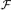(N, b, z)dN db dz, then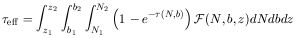(2)

(Paresce et al 1980), for a population of absorbers without spatial or velocity correlations. Assuming that the N and b distribution functions are independent of redshift, and the redshift evolution of the number density of lines can be approximated by a power law, we can write(N, b, z) = (1 + z)F(N, b), and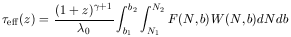(3)

where the rest frame equivalent width is given by W = (1 + z)-1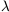0-1d(1 - e-).

This relation enables us to measure the redshift evolution of the number density of Lyforest clouds, d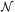/ dz(1 + z), from the redshift dependence of the effective optical depth (eff(1 + z)+1) even if we do not resolve the individual absorption lines (Young et al 1982b; Jenkins & Ostriker 1991; Zuo 1993; Press et al 1993; Lu & Zuo 1994). The results of this approach are discussed below in the section on Lyforest evolution, together with the conclusions from line counting methods.

The largest uncertainties in D oreff are probably caused by our ignorance about the precise QSO continuum level, against which the absorption is measured. Additional errors stem from the amount of absorption contributed by metal lines, which often cannot be identified as such and then removed from low resolution Lyspectra.